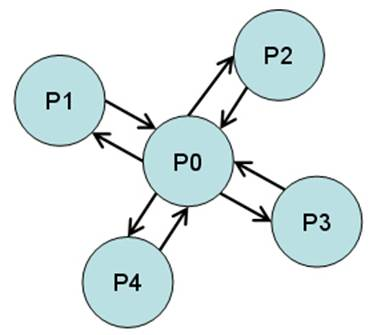SE 765 - Distributed Software Development CS 610 - Introduction to Parallel and Distributed Computing

Assignment #4

Note:

·           All programs must be your own work.

·           As with Assignment #2 send the .erl and a screen capture of each program executing to me.

·           Due date: 6 PM, Monday, November 24, 2014

Exercise 1

Modify tut16 from the 3rd Erlang lecture to start N + 1 processes in a star, and sends a message M times forwards and backwards between the center process and the other processes. After all the messages sent the processes should terminate gracefully.The output of the function should look something similar to the following (N = 3, M = 4):

> procs:star(3, 4).

Current process is <0.31.0>

Created <0.33.0> (center)

Created <0.34.0>

Created <0.35.0>

Created <0.36.0>

<0.34.0> finished

<0.35.0> finished

<0.36.0> finished

<0.33.0> finished

Exercise 2

Requirements: A computer with a dual (or better) core processor, such as an Intel Centrino Duo or an AMD Turion 64 X2. Actually, any Erlang supported symmetric multiprocessing (SMP) computer system should work. An SMP computer has two or more identical CPUs that are connected to a single shared memory. These CPUs may be on a single multi-core chip, spread across several chips, or a combination of both.

For this experiment two different problem sets will be used:

·          Test 1: Sort 100 lists, each one with 1,000 random integers.

·          Test 2: Compute 100 times the 27th element of the Fibonacci sequence.

Each test will be executed using two different versions of the map function: the ordinary (sequential) lists:map and the parallel plists:map. By default, this last function operates over each list element using an independent Erlang process.

NOTE: In order to use the plists module, extract plists-1.0.zip, copy the plists-1.0/src/plists.erl file to your working directory and compile it.

For Test 1, a function must create a list with K random integers in the range 1...1,000,000. The following code achieves this:

make_random_list(K) -> [random:uniform(1000000) || _ <- lists:duplicate(K, null)].

The seed function resets the random number generator. This is so the same sequence of random numbers is generated each time we run the program:

seed() -> random:seed(44,55,66).

This code creates a list L1 with 100 lists containing 1,000 random integers each:

L1 = [make_random_list(1000) || _ <- lists:duplicate(100, null)].

For Test 2, a function must compute the n-th element of the Fibonacci sequence. The following computational intensive (and inefficient) recursive definition will be used:

fibo(0) -> 1;

fibo(1) -> 1;

fibo(N) -> fibo(N-1) + fibo(N-2).

The list L2, containing 100 twenty-sevens, can be defined as:

L2 = lists:duplicate(100, 27).

In summary, the objective of the experiment is to measure the time it takes to execute the four expressions contained in the following table:

 Expression Category Description lists:map(fun lists:sort/1, L1) Sequential Light-weight computations with a lot of message passing: Sort 100 lists, each one with 1,000 random integers. plists:map(fun lists:sort/1, L1) Parallel lists:map(fun fibo/1, L2) Sequential CPU-bound computations with little message passing: Compute 100 times the 27th element of the Fibonacci sequence. plists:map(fun fibo/1, L2) Parallel

In order to measure the execution time, the timer:tc function will be used, which takes three parameters: Module, FunName, and ArgList. It calls the corresponding function with the specified arguments and measures the elapsed real time. It returns a tuple {Time, Value}, where Time is the elapsed real time in microseconds, and Value is what the function returns. For example:

> {T,V} = timer:tc(lists,seq,[1,20]).

{1,[1,2,3,4,5,6,7,8,9,10,11,12,13,14,15,16,17,18,19,20]}

> T.

1

> V.

[1,2,3,4,5,6,7,8,9,10,11,12,13,14,15,16,17,18,19,20]

Once time measurements have been collected, the speedup can be calculated, which refers to how much a parallel program is faster than a corresponding sequential program.

The speedup is defined by the following formula:

Sp=T1÷ Tp

Where:

·         p is the number of processors.

·         T1 is the execution time of the sequential program.

·         Tp is the execution time of the parallel program with p processors.

Everything is put together in a file called multicore.erl:

-module(multicore).

-export([test1/0,test2/0]).

make_random_list(K) -> [random:uniform(1000000) || _ <- lists:duplicate(K, null)].

seed() -> random:seed(44,55,66).

fibo(0) -> 1;

fibo(1) -> 1;

fibo(N) -> fibo(N-1) + fibo(N-2).

test1() ->

seed(),

L1 = [make_random_list(1000) || _ <- lists:duplicate(100, null)],

{Time1, S1} = timer:tc(lists, map, [fun lists:sort/1, L1]),

{Time2, S2} = timer:tc(plists, map, [fun lists:sort/1, L1]),

io:format("sort: results equal? = ~w, speedup = ~w~n", [S1=:=S2, Time1/Time2]).

test2() ->

L2 = lists:duplicate(100, 27),

{Time1, S1} = timer:tc(lists, map, [fun fibo/1, L2]),

{Time2, S2} = timer:tc(plists, map, [fun fibo/1, L2]),

io:format("fibo: results equal? = ~w, speedup = ~w~n", [S1=:=S2, Time1/Time2]).

Compile the module and run the functions test1 and test2.  Which of the two tests has the best speedup? Why do you think this is so?

By default, the Erlang runtime system starts with SMP support enabled if it's available and two or more logical processors are detected.

When you launch the Erlang shell, you can specify the "+S N" option to indicate that N schedulers should be used (each Erlang scheduler is a complete virtual machine that knows about all the other virtual machines). For example, the following command indicates that only one scheduler should be used:

\$ erl +S 1

NOTE: In Windows, open a command window (cmd.exe) and use the werl command instead (normally found in the C:\Program Files\erl5.6.1\bin directory) supplying the same +S option.

If the +S option is omitted, it defaults to the number of logical processors in the SMP machine.

Run again test1 and test2, but this time using only one scheduler. What happens to the speedups? Why do you think this happens?

Finally, run test1 and test2, but now using more schedulers than the number of logical processors available. What can you conclude from the observed results?